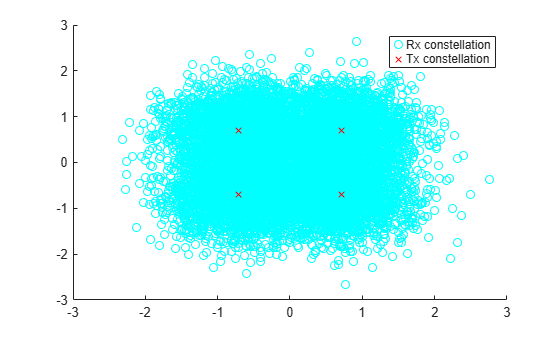# lteTurboDecode

Turbo decoding

## Syntax

``out = lteTurboDecode(in)``
``out = lteTurboDecode(in,nturbodecits)``

## Description

example

````out = lteTurboDecode(in)` returns the result of turbo decoding the input data `in`. The function can decode single data vectors or cell arrays of data vectors. In the case of cell array input, the output is a cell array containing the separately decoded input array vectors. The input data is assumed to be soft bit data that has been encoded with the parallel concatenated convolutional code (PCCC), as defined in TS 36.212 , Section 5.1.3.2. Each input data vector is assumed to be structured as three encoded parity streams concatenated in a block-wise fashion, `[S P1 P2]`, where `S` is the vector of systematic bits, `P1` is the vector of encoder 1 bits, and `P2` is the vector of encoder 2 bits. The decoder uses a default value of 5 iteration cycles. It returns the decoded bits in output vector `out` after performing turbo decoding using a sub-log-MAP (Max-Log-MAP) algorithm. ```
````out = lteTurboDecode(in,nturbodecits)` provides control over the number of turbo decoding iteration cycles via parameter `nturbodecits`. The `nturbodecits` is an optional parameter. If it is not provided, it uses the default value of 5 iteration cycles. ```

## Examples

collapse all

Perform turbo decoding of soft bits obtained from a noisy constellation.

Create a bit stream, turbo encode the bit stream, and modulate it. Create noise, add it to the modulated symbols. Display the transmitted and received symbols on a scatter plot.

```txBits = randi([0 1],6144,1); codedData = lteTurboEncode(txBits); txSymbols = lteSymbolModulate(codedData,'QPSK'); noise = 0.5*complex(randn(size(txSymbols)),randn(size(txSymbols))); rxSymbols = txSymbols + noise; scatter(real(rxSymbols),imag(rxSymbols),'co'); hold on; scatter(real(txSymbols),imag(txSymbols),'rx') legend('Rx constellation','Tx constellation')```Demodulate the symbols and turbo decode soft bits. Compare the transmitted and recovered bits.

```softBits = lteSymbolDemodulate(rxSymbols,'QPSK','Soft'); rxBits = lteTurboDecode(softBits); numberErrors = sum(rxBits ~= int8(txBits))```
```numberErrors = 0 ```

## Input Arguments

collapse all

Soft bit input data, specified as a numeric vector or a cell array of vectors. The decoder expects the input bits to be encoded with the parallel concatenated convolutional code (PCCC), as defined in TS 36.212 , Section 5.1.3.

Data Types: `int8` | `double` | `cell`

Number of turbo decoder iteration cycles, specified as a positive scalar integer between 1 and 30. Optional.

Data Types: `double`

## Output Arguments

collapse all

Turbo decoded bits, returned as an integer column vector or a cell array of integer column vectors.

Data Types: `int8` | `cell`

 3GPP TS 36.212. “Evolved Universal Terrestrial Radio Access (E-UTRA); Multiplexing and channel coding.” 3rd Generation Partnership Project; Technical Specification Group Radio Access Network. URL: https://www.3gpp.org.